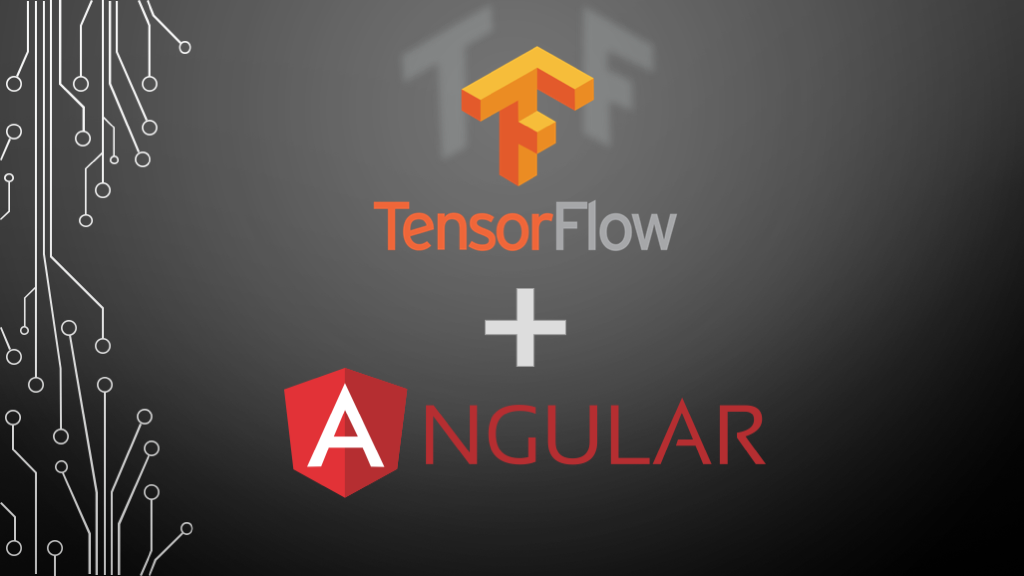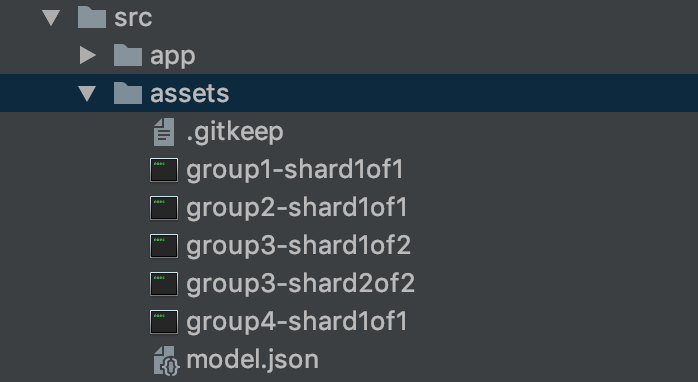Goalist Developers Blog

# Using pre-trained Machine Learning (ML) Models in the browser with TensorFlow.js & Angular

Greetings for the day! My name is Vivek.

In this blog post, let's see how to use your pre-trained Machine Learning (ML) model directly in the browser using Tensorflow.js and AngularThe following section of this blog is interactive, so you can try to draw a number between 0 ~ 9 and see the predicted output in the browser⤵︎
Go ahead and try it yourself, draw a number inside this blue box↓

Amazzing isn't it? Let's learn how to do this step by step

TensorFlow for Javascript has a Python CLI tool that converts an h5 model saved in Keras to a set of files that can be used on the web.
To install it, run the following command

`pip install tensorflowjs`

At this point, you will need to have a Keras model saved on your local system.

Suppose you have your Keras Model save at the following location
input_path/file_name.h5
and suppose you want to generate output at the following location
path_to_output_folder/
In that case your command to convert model will look something like this

```tensorflowjs_converter --input_format keras \
input_path/file_name.h5 \
path_to_output_folder
```

In my case, the model is located in keras/cnn.h5 and I would like to keep my converted model at src/assets directory so I shall run the following command

```tensorflowjs_converter --input_format keras \
keras/cnn.h5 \
src/assets
```

Input and Output directories should look similar to this
InputOutputTo load the model, you need TensorFlow.js library in your Angular application
Install it using Node Package Manager

`npm install @tensorflow/tfjs --save`

```import {Component, OnInit} from '@angular/core';
import * as tf from '@tensorflow/tfjs';

@Component({
selector: 'app-root',
templateUrl: './app.component.html',
styleUrls: ['./app.component.scss'],
})
export class AppComponent implements OnInit {

model: tf.Model;

ngOnInit() {
}

}

}
```

### #Step 3) Make predictions using live drawn image data in the browser

Now that our model is loaded, it is expecting 4-dimensional image data in a shape of
[any, 28, 28, 1]
[batchsize, width pixels, height pixels, color channels]

Just trying to avoid memory leaks and to clean up the intermediate memory allocated to the tensors we run our predictions inside of `tf.tidy()` ( TensorFlow.js)

TensorFlow.js gives us a fromPixels (TensorFlow.js) helper to convert an ImageData HTML object into a Tensor.
So the complete code looks like this ↓

```import {Component, OnInit} from '@angular/core';

import * as tf from '@tensorflow/tfjs';

@Component({
selector: 'app-root',
templateUrl: './app.component.html',
styleUrls: ['./app.component.scss'],
})
export class AppComponent implements OnInit {

model: tf.Model;
predictions: any;

ngOnInit() {
}

}

// Do predictions
async predict(imageData: ImageData) {

const pred = await tf.tidy(() => {

// Convert the canvas pixels to
let img = tf.fromPixels(imageData, 1);
// @ts-ignore
img = img.reshape([1, 28, 28, 1]);
img = tf.cast(img, 'float32');

// Make and format the predications
const output = this.model.predict(img) as any;

// Save predictions on the component
this.predictions = Array.from(output.dataSync());
});

}

}
```

And component HTML looks like this

```<div class="container">

<!--Input Section-->
<div class="column justify-content-center">
<div class="col-sm">
<h5>Draw a number here </h5>
<div class="wrapper">
<canvas drawable (newImage)="predict(\$event)"></canvas>
<br>
</div>
<button class="btn btn-sm btn-warning" (click)="canvas.clear()">Erase</button>
</div>

<!--Prediction Section-->
<div class="col-sm predict">
<h5>TensorFlow Prediction</h5>
<chart [data]="predictions"></chart>
</div>
</div>
</div>

<router-outlet></router-outlet>
```

There we go... we just used Machine Learning in the browser.